> Example: Drawing a rectangle using a line between two points as a reference

Example: Drawing a rectangle using a line between two points as a reference
When you draw 2D geometrical entities using the 2D CoPilot, you can use the Line Between 2 Points command (on-the-fly) to create a temporary line, and then use the midpoint of this line as a reference to create geometrical entities. For example, the following image shows a rectangle which passes through the midpoints of the diagonals of two other rectangles.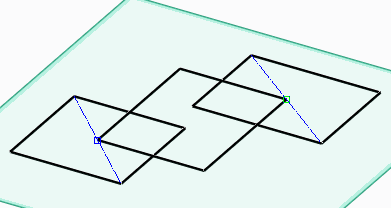To draw the above rectangle:
1. Select a workplane in the viewport and click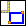on the Command Mini Toolbar (CMT).
2. Press M or right-click and choose Line Between 2 Points on the context menu.
Alternatively, press SPACEBAR or the assigned key and click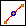on the Option Mini Toolbar (OMT).
3. Select the two opposite vertices of the first rectangle to draw the first diagonal.4. Select the midpoint of the first diagonal to mark the first corner of the rectangle and move the cursor.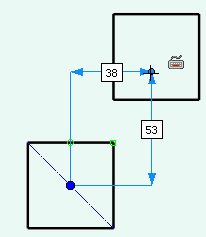5. Press M and select the two opposite vertices of the second rectangle as shown below, to draw the second diagonal.6. Select the midpoint of the diagonal to mark the second corner of the rectangle.7. Click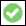to complete the operation. The temporary diagonals disappear.